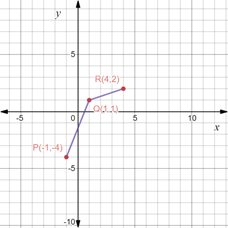# The coordinates of the point S so that quadrilateral PQRS is a parallelogram.### Precalculus: Mathematics for Calcu...

6th Edition
Stewart + 5 others
Publisher: Cengage Learning
ISBN: 9780840068071### Precalculus: Mathematics for Calcu...

6th Edition
Stewart + 5 others
Publisher: Cengage Learning
ISBN: 9780840068071

#### Solutions

Chapter 1.8, Problem 45E
To determine

## To calculate: The coordinates of the point S so that quadrilateral PQRS is a parallelogram.

Expert Solution

The coordinates of point S so that PQRS forms a parallelogram is S(2,3) .

### Explanation of Solution

Given information:

The points P(1,4),Q(1,1) and R(4,2) .

Formula used:

A parallelogram is a quadrilateral in which diagonals bisect each other at the same point.

Mid-point formula between two points X(a1,b1) and Y(a2,b2) is mathematically expressed as,

(a1+a22,b1+b22)

Calculation:

Consider the provided vertices P(1,4),Q(1,1) and R(4,2) .

By plotting the given points on the coordinate plane, we get the following figure,Recall that a parallelogram is a quadrilateral in which diagonals bisect each other at the same point.

So, to find the coordinates of S such that PQRS forms a parallelogram, the mid-point of its diagonals i.e. PR and QS must be equal.

Let the coordinates of the point be (a,b) .

Recall that the mid-point formula between two points X(a1,b1) and Y(a2,b2) is mathematically expressed as,

(a1+a22,b1+b22)

So, midpoint of PR is calculated as,

(1+42,4+22)=(32,22)=(32,1)

Now, midpoint of QS is calculated as,

(1+a2,1+b2)

Since, diagonals of a parallelogram bisect each other at same point, so midpoint of PR and QS is same.

So, equate the mid-points of PR and QS as,

(1+a2,1+b2)=(32,1)

Now, equate x-coordinate and y-coordinate from both sides of the equation as,

1+a2=321+a=3a=31a=2

1+b2=11+b=2b=21b=3

Thus, the coordinates of point S so that PQRS forms a parallelogram is S(2,3) .

### Have a homework question?

Subscribe to bartleby learn! Ask subject matter experts 30 homework questions each month. Plus, you’ll have access to millions of step-by-step textbook answers!